• Power Calculations
 • Relative Prediction Variance
 • Power for a Single Parameter
 • Power for a Categorical Effect
The model matrix. See the Fitting Linear Models book for information on the coding for nominal effects. Also, see The Model Matrix in Technical Details.
Note: You can view the model matrix by running Fit Model. Then select Save Columns > Save Coding Table from the red triangle menu for the main report.
The variance of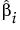is given by the ith diagonal entry of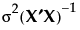, where σ2 is the error variance. Denote the ith diagonal entry of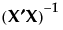by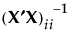.
The error variance, σ2, is estimated by the MSE, and has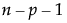degrees of freedom, where n is the number of observations and p is the number of terms other than the intercept in the model.
Under the null hypothesis, the test statistic F0 has an F distribution on 1 anddegrees of freedom.
 X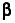Vector of parameters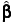Least squares estimate of β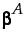Vector of Anticipated Coefficient values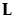Matrix that defines the test for the categorical effect. The matrix L identifies the values of the parameters in β corresponding to the categorical effect and sets them equal to 0. The null hypothesis for the test of the categorical effect is given by: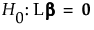r Rank of L. Alternatively, r is the number of levels of the categorical effect minus one.
Note: You can view the design matrix by running Fit Model. Then select Save Columns > Save Coding Table from the red triangle menu for the main report.
The error variance, σ2, is estimated by the MSE, and hasdegrees of freedom, where n is the number of observations and p is the number of terms other than the intercept in the model.
Under the null hypothesis, the test statistic F0 has an F distribution on r anddegrees of freedom.
If the true value of β is, then F0 has a noncentral F distribution with noncentrality parameter given by:
 X σ2 Error varianceVector of least squares estimates of the parameters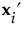The ith row of X